# Correlations, Partial Correlations, and Confounding Variables

In this post we discuss the calculation of the correlation coefficient between two variables, X and Y, and the partial correlation coefficient which controls for the effect of a potential confounding variable, Z

When we take the correlation of two variables, X and Y, one is usually referred to as the independent variable (say X), and one is usually referred to as the dependent variable (say Y is the dependent variable).  This nomenclature indicates an a priori assumption that variable Y depends on variable X in some way (ie; in some fashion, variation in X causes variation in Y).

Sometimes, however, one or more confounding variables (aka: confounding factor, lurking variable, or confounder) exist that make it appear that Y is related to X, but the relationship is in fact spurious.

An example is the statistical relationship between ice cream sales and drowning deaths per month over time. When these variables are entered into a statistical analysis, they will show a positive and potentially statistically significant correlation. However, it would be a mistake to infer a causal relationship (i.e., ice cream causes drowning) , because of the presence of an important confounding variable which causes both ice cream sales and an increase in drowning deaths: summertime.

A further example will be seen in the Analysis Hall of Shame, where a group of researchers failed to take into account (some rather obvious) confounding variables, and drew what is almost certainly a faulty conclusion about a casual relationship between two variables.

How can we take into account confounding variables?  We will see when we get to the linear regression model that it is easy to include the effect of multiple confounders in a regression analysis.  In an analysis of correlation, however, confounders are taken into account by taking partial correlations; for instance, the partial correlation of X and Y taking potential confounder Z into account is the correlation of X and Y after any relationship to Z has been corrected for.

It is very important to note here that correlation does not imply causation! Thus, even after taking into account a potential confounding variable, if X and Y still appear to be significantly correlated there may exist yet another confounding variable underlying that apparent relationship.  Tyler Vigen has a humorous website and book devoted to spurious correlations.  There has been a purported relationship between the use of leaded gasoline over time, and crime rates.  Given what you saw on Tyler Vigen’s website, what are your thoughts on this purported relationship?  What ways could we be more certain that the relationship is causal?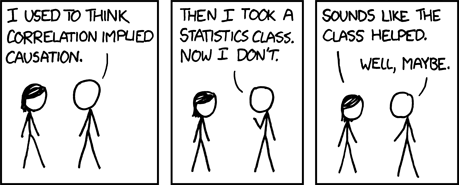However, finding that X and Y that are significantly correlated, even after taking into account obvious confounding variables, can be a compelling reason to design research studies to determine if there is evidence of causality. A good example of this is noting a correlation between the time series of deaths due to heart disease, and the time series of the fraction of people who smoke over time.    It was noted many years ago that a significant correlation exists between these two time series.  But does smoking cause heart disease?  To answer that question, a complicated study had to be designed where groups of people were followed over several years, taking note of whether or not they smoked, how much they smoked, and for how many years they smoked.  The incidence of heart disease in people in the group was assessed, and its correlation to smoking habits, taking into account socio-economic and demographic factors.  The conclusion is that, with a high degree of statistical significance, smoking likely causes heart disease.

Calculation of correlation and partial correlation statistics

The Pearson linear correlation coefficient between X and Y is often denoted as r, or also as rho, or rho_XY.  The formula is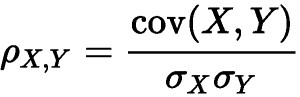where N is the size of the sample, and S_X and S_Y are the X and Y sample standard deviations. Note that rho_XY=rho_YX.  The R function cor(x,y) calculates the correlation between x and y.  The Vassar statistics correlation coefficient significance calculator page is a handy online tool for calculating the p-value that tests the null hypothesis that rho_XY is consistent with zero.  The R cor.test() function also does this within R.

The test used by the Vassars stat page and the cor.test() function is the Fishers Z-transformation significance test, which assumes that X and Y are Normally distributed. If they aren’t, then applying the test can lead to incorrect p-value assessment when testing the null hypothesis. The Spearman rho correlation coefficient helps to fix this, by first mapping the X and Y data onto a Normal distribution using a rank-Normal transformation, then calculating the correlations between the transformed variables. In R, you can obtain the Spearman rho correlation coefficient by using the method=”spearman” option in the cor() and cor.test() methods. Here’s how a rank-Normal transformation works:Example

The file exam_anxiety.csv contains data on student exam scores from the book “Discovering Statistics Using R” by Field, Miles, and Field, along with a measure of their anxiety before they took the exam, and how long they studied for the exam.  The R script exam_anxiety.R reads in this file, and produces the following plot: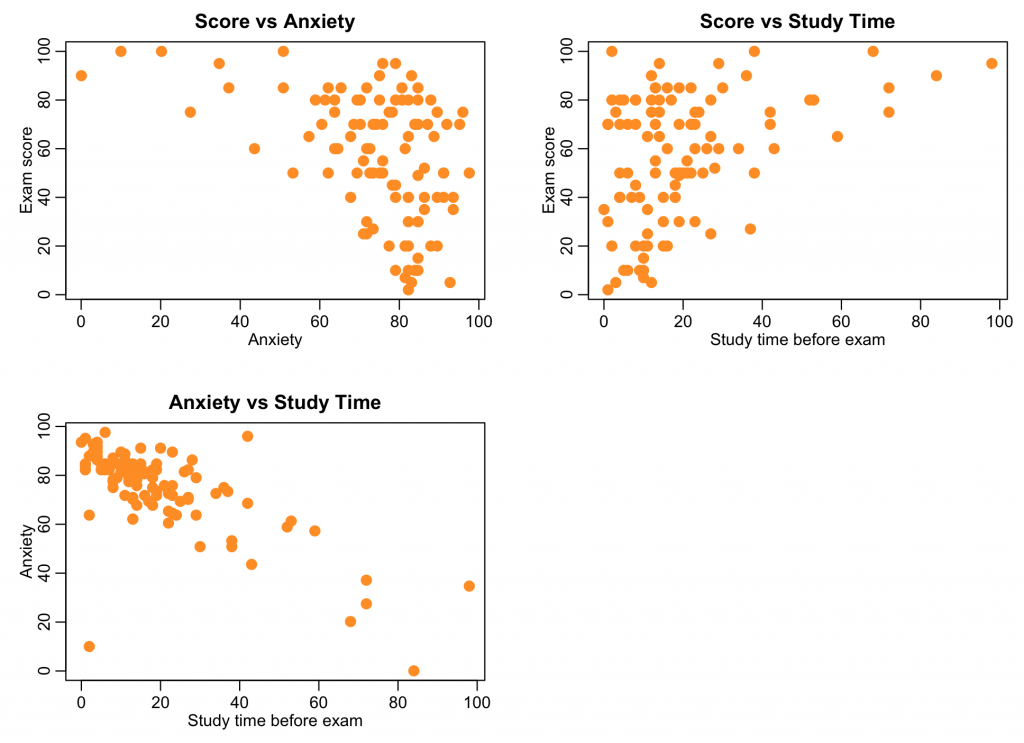There appears to be a potential relationship between anxiety and study time, and exam performance.

When we histogram the data, however, it is clear that some of these variables are not Normally distributed: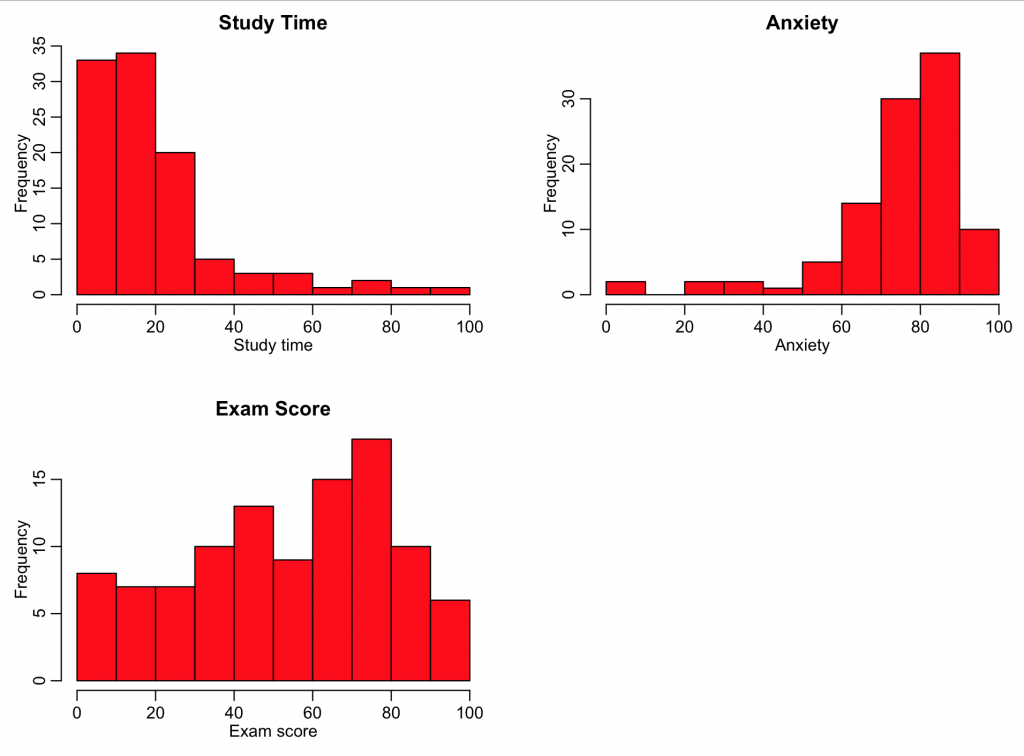Thus, for these data, we should use the Spearman correlation coefficient. The R script uses the cor.test() function in R to test the statistical significance of the correlations.

Partial correlation coefficient

The partial correlation of X and Y, taking into account Z is rho_XY|Z.  The formula is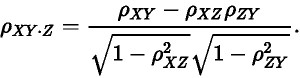In R, the pcor.test(x,y,z) function in the ppcor library calculates the correlation between x and y, taking into account z. It also gives the p-value testing the null hypothesis that the true partial correlation is consistent with zero.  Alternatively, to calculate the p-value go to the Vassar correlation significance calculator page, and enter rho_XY|Z into the field for r, and enter the size of your sample, minus 1, into the field for N.

The R script exam_anxiety.R calculates the partial correlation of exam scores with anxiety, controlling for study time, and also the partial correlation of exam scores with study time, controlling for anxiety, using the Spearman correlation coefficient: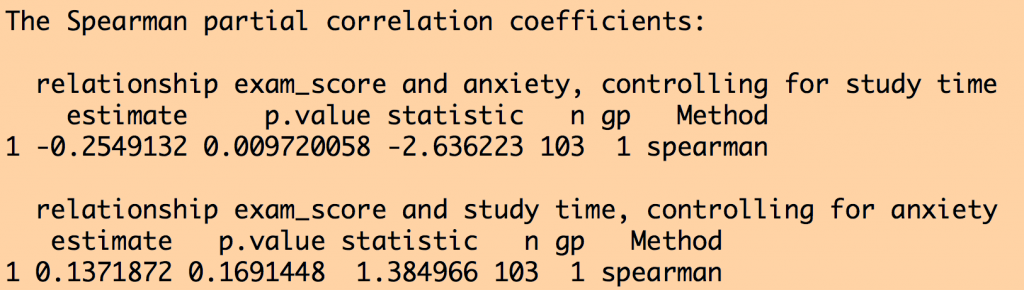It turns out that once study time is accounted for exam scores are significantly anti-correlated to anxiety.  However, once anxiety is accounted for, exam scores are no longer significantly correlated to study time.  That doesn’t mean that study time doesn’t matter, by the way… the study was only based on 103 students, which is a relatively small sample size.

Visits: 11517